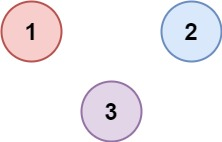# Number of Provinces Leetcode Solution

Difficulty Level Medium
ByteDancViews 3589

## Problem Statement

Number of Provinces Leetcode Solution – We are given an adjacency matrix representation of a graph and need to find the number of provinces. Here province is a group of directly or indirectly connected cities and no other cities outside of the group.

## Example

Example 1:```Input: isConnected = [[1,1,0],[1,1,0],[0,0,1]]
Output: 2```

Example 2:```Input: isConnected = [[1,0,0],[0,1,0],[0,0,1]]
Output: 3```

## Explanation for Number of Provinces Leetcode Solution

• In the first eg. we can see there is an edge between 1 & 2, hence they are directly connected and counted as a single province. Node 3 is isolated and counted as a separate province. Therefore, there are 2 provinces.
• In the 2nd eg. None of them have any edge and hence 3 provinces

## Approach

We can use the DFS algorithm to traverse all the nodes directly or indirectly connected to a specific node. We will run DFS only on the nodes that are not visited. Hence, this question boils down to finding the number of disconnected components in a graph. The number of times DFS runs will give us the number of provinces.

## Code

### C++ code for Number of Provinces

```class Solution {
void dfs(vector<vector<int>> &isConnected, vector<int> &vis, int u, int nodes) {
// cout<<u<<" ";
vis[u]=1;
for(int v=0; v<nodes; v++) {
if(u!=v && isConnected[u][v]==1) {
if(!vis[v]) {
dfs(isConnected, vis, v, nodes);
}
}
}
}
public:
int findCircleNum(vector<vector<int>>& isConnected) {
int nodes = isConnected.size();
vector<int> vis(nodes, 0);
int cnt=0;
for(int i=0; i<nodes; i++) {
if(!vis[i]) {
cnt++;
dfs(isConnected, vis, i, nodes);
}

}

return cnt;
}
};```

### Java code for Number of Provinces

```class Solution {
public void dfs(int[][] isConnected, boolean[] vis, int u, int nodes) {
vis[u]=true;
for(int v=0; v<nodes; v++) {
if(u!=v && isConnected[u][v]==1) {
if(!vis[v]) {
dfs(isConnected, vis, v, nodes);
}
}
}

}
public int findCircleNum(int[][] isConnected) {
int nodes = isConnected.length;
boolean [] vis = new boolean[nodes];
int cnt = 0;
for(int i=0; i<nodes; i++) {
if(!vis[i]) {
cnt++;
dfs(isConnected, vis, i, nodes);
}

}
return cnt;
}
}```

## Complexity Analysis for Number of Provinces LeetCode Solution

• Time complexity: O(n^2)
• The complete matrix (isConnected) of size n^2 is traversed.
• Space complexity: O(n)
• a visited array (vis) of size n is used.
Translate »